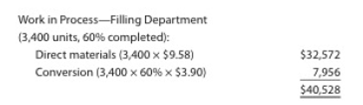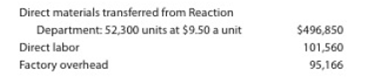Chapter 20, Problem 20.3BPR

Chapter
Section
Textbook Problem

Equivalent units and related costs; cost of production report; entriesDiner Chemical Company manufactures specialty specials by a series of three processes, all materials being introduced in the Distilling Department. From the Distilling Department, the materials pass through the Reaction and Filling departments, emerging as finished chemicals.The balance in the account Work in Process—Filling was as follows on January 1:The following costs were charged to Work in Process—Filling during January:During January, 53,000 units of specially chemicals were completed. Work in Process—Filling Department on January 31 was 2,700 units, 30% completed.Instructions1. Prepare a cost of production report for the Filling Department for January.2. Journalize the entries for costs transferred from Reaction to Filling and the costs transferred from Filling to Finished Goods.3. Determine tile increase or decrease in the cost per equivalent unit from December to January for direct materials and conversion costs.4. Discuss the uses of the cost of production report and the results of part (3).

(1)

To determine

Process costs

It is a method of cost accounting, which is used where the production is continuous, and the product needs various processes to complete. This method is used to ascertain the cost of the product at each process or stage of production.

Equivalents units for production

The activity of a processing department in terms of fully completed units is known as equivalent units. It includes the completed units of direct materials and conversion cost of beginning work in process, units completed and transferred out, and ending work in process.

Production cost report

A production cost report is a comprehensive report prepared for each department separately at the end of a particular period, which represents the physical flow and cost flow of product for the concerned department.

To Determine: The equivalents units for production of direct materials and conversion costs for the month of July for Company DC.

Explanation

Calculate the equivalents units for production of direct materials and conversion costs for the month of July for Company DC as shown below:

Figure (1)

Working notes:

Calculate opening work in process inventory for conversion costs as shown below:

Opening work in process inventory for conversion costs) = 160%=40%

Calculate units started and completed in Januay as shown below:

Units started and completed in January = (Units transferred out opening  work in process inventory)=53,000units 3,400units=49,600units

Calculate ending work in process inventory for conversion costs as shown below:

Ending work in process inventory for conversion costs) = (Total units for ending work in proces inventory ×Completed percent for conversion costs)=2,700units ×30%=810units

Equivalent units for production is calculated by adding units of opening work in process inventory, transferred to finished goods in July, and units for ending work in process inventory. Therefore, an equivalents unit for production for direct materials is 52,300 units and equivalent units for production for conversion costs is 51,770 units.

Calculate direct materials and conversion cost equivalent cost per unit for January as shown below:

Direct material cost per unit =Total direct material costsEquivalent units for direct materials=$496,85052,300units=$9.50Per unit

Conversion cost per unit =Total conversion costsEquivalent units for conversion=$196,72651,770units=$3.80Per unit

Working note:

Calculate total conversion cost for the month of January for Company DC as shown below:

Total conversion cost = Direct labor cost+Factory overhead cost=$101,560+$95,166=\$196,726

Direct material cost per unit is calculated by dividing total direct materials cost by equivalent units for direct materials

(2)

To determine

To Prepare: The journal entry to record cost transferred from reaction department to filling department and cost transferred from filling department to finished goods during the month of January.

(3)

To determine
Increase or decrease in the cost per equivalent per unit for direct materials and conversion cost between December and January.

(4)

To determine

To Discuss: The uses of cost of production report.

Still sussing out bartleby?

Check out a sample textbook solution.

See a sample solution

The Solution to Your Study Problems

Bartleby provides explanations to thousands of textbook problems written by our experts, many with advanced degrees!

Get Started

Explain the matching principle.

College Accounting, Chapters 1-27

In what ways is economics a science?

Essentials of Economics (MindTap Course List)

Define an internal transaction and provide an example.

College Accounting (Book Only): A Career Approach

If a typical firm reports 20 million of retained earnings on its balance sheet, could its directors declare a 2...

Fundamentals of Financial Management, Concise Edition (with Thomson ONE - Business School Edition, 1 term (6 months) Printed Access Card) (MindTap Course List)